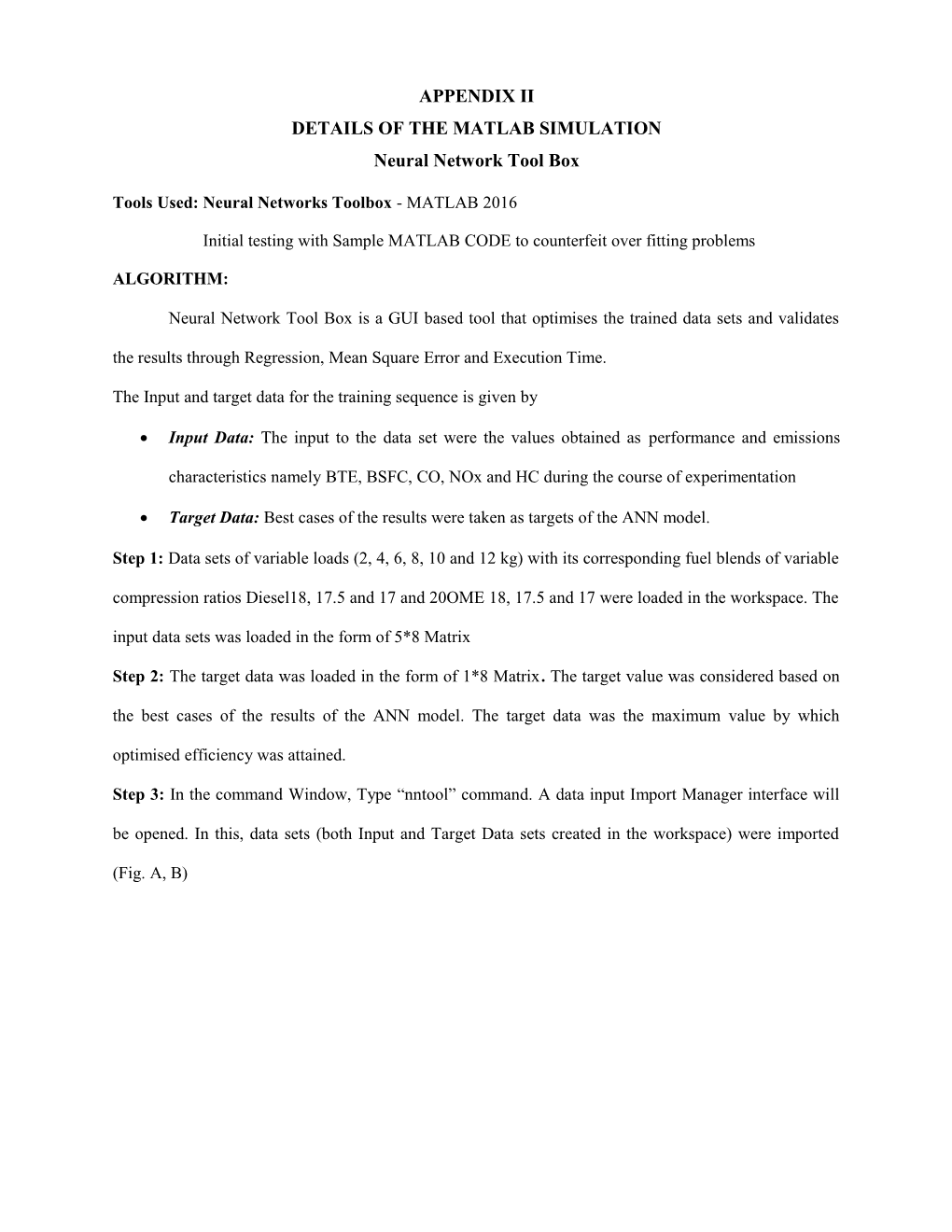# Details of the Matlab SimulationAPPENDIX II

DETAILS OF THE MATLAB SIMULATION

Neural Network Tool Box

Tools Used: Neural Networks Toolbox- MATLAB 2016

Initial testing with Sample MATLAB CODE to counterfeit over fitting problems

ALGORITHM:

Neural Network Tool Box is a GUI based tool that optimises the trained data sets and validates the results through Regression, Mean Square Error and Execution Time.

The Input and target data for the training sequence is given by

• Input Data:The input to the data set were the values obtained as performance and emissions characteristics namely BTE, BSFC, CO, NOx and HC during the course of experimentation
• Target Data:Best cases of the results were taken as targets of the ANN model.

Step1: Data sets of variable loads (2,4,6,8,10 and 12 kg) with its corresponding fuel blends of variable compression ratios Diesel18,17.5 and17 and 20OME 18,17.5 and 17 were loaded in the workspace. The input data sets was loaded in the form of 5*8 Matrix

Step2:The target data was loaded in the form of 1*8 Matrix. The target value was considered based on thebest cases of the results of the ANN model. The target data was the maximum value by which optimised efficiency was attained.

Step3:In the command Window, Type “nntool” command. A data input Import Manager interface will be opened. In this, data sets (both Input and Target Data sets created in the workspace) were imported (Fig.A,B)Fig. A.NNtool WindowFig.B.Import to Data Manager

Step 4: Neural Network Data/Manager was loaded. The Network was created by specifying

Network Type:

Input Data:

Target Data:

Training Function:

Number of Neurons:

Transfer Function:Fig.C.Creation of Network Window

On specification, Create Network button is clicked in the GUI and Pop UP displaying “Network is created” will be visible in the screen.Fig.D.Creation of Network Popup Window

Step5: Once the network was created, Training of networks with the data sets was done bycategorizing the randomized 6 samples of 5 elements into 70% (4 Samples) for training, 15% (1 Sample) for validation and 15% (1 sample) for testing and subjected for the following training specifications

Show:25 Epochs -10000, lr-0.001

Minimum gradient 1*10-7 Max fail of the targeted values at 1000 (Maximum iterations).Fig.E.Training Parameters initialisation

ANN model starts with the process of fitting the inputs and target data. The input and target fitting problem decays on setting the randomly divide up of the samples for validation and testing.Fig.F.Training of Data

SAMPLE MATLAB CODE

x = data;%% Assigning Variable to Input data.

t = target;%% Assigning Variable to target data.

% %Choose of Training Function

%% For a list of all training functions type as mentioned in Table-6

trainFcn = 'trainlm'; % training network Trainlm

%% Create a Feedforward Network

hiddenLayerSize = 18; %% Defining the hidden layer

net = feedforwardnet (hiddenLayerSize,trainFcn); %% Inbuilt Function of Feed Forward Network with the variables called

%% Setup Division of Data for Training, Validation, Testing

RandStream.setGlobalStream(RandStream('mt19937ar','seed',1)); % to get constant result

net.divideFcn = 'divideblock'; %% Divide targets into three sets using blocks of indices

net.divideParam.trainRatio = 70/100;

net.divideParam.valRatio = 15/100;

net.divideParam.testRatio = 15/100;

%TRAINING PARAMETERS

net.trainParam.show=10000; % %# of ephocs in display

net.trainParam.lr=0.001; %%learning rate

net.trainParam.epochs=10000; % %max epochs

net.trainParam.goal=1000; % %training goal

net.performFcn='mse'; % %Name of a network performance function %type help nnperformance

%% Train the Network

[net,tr] = train(net,x,t);

% Testingof Network

y = net(x);

e = gsubtract(t,y);

performance = perform(net,t,y)

% View the Network

view(net)